## Determination of the molecular weight of polymers by end-group analysis

One of the main parameters determining the properties of a polymer is the degree of polymerization which is directly related to the molecular weight. The degree of polymerization (DP), or number of repeating units, can be determined by comparing in the 1D 1H spectra the integral of the end-group and the integral of the repeating chain unit of interest by using this equation: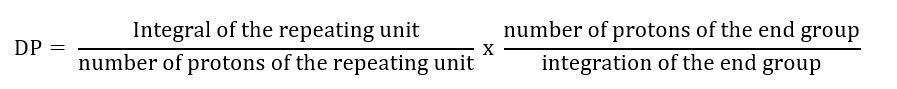The molecular weight of the polymer can then easily be determined by multiplying the DP with the molecular weight of the repeating unit.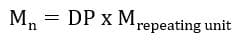Typically, the molecular weight of the end group is not taken into consideration for the calculation as it is usually negligible compared to the total molecular weight of the polymer chains.

The first graph shows a 1H spectrum measured on Spinsolve 80 MHz system of a methoxy terminated polyethylene glycol (PEG) spectrum. This polymer, also known as polyethylene oxide, is commercially available and finds applications in pharmaceutical, medical, cosmetics, and food. The strong signal at 3.7 ppm originates from the CH2 groups, while the signal at 3.4 ppm originates from the methoxy end group. The two signals can be integrated and the degree of polymerization as well the molecular weight can be obtained by using the equations described above.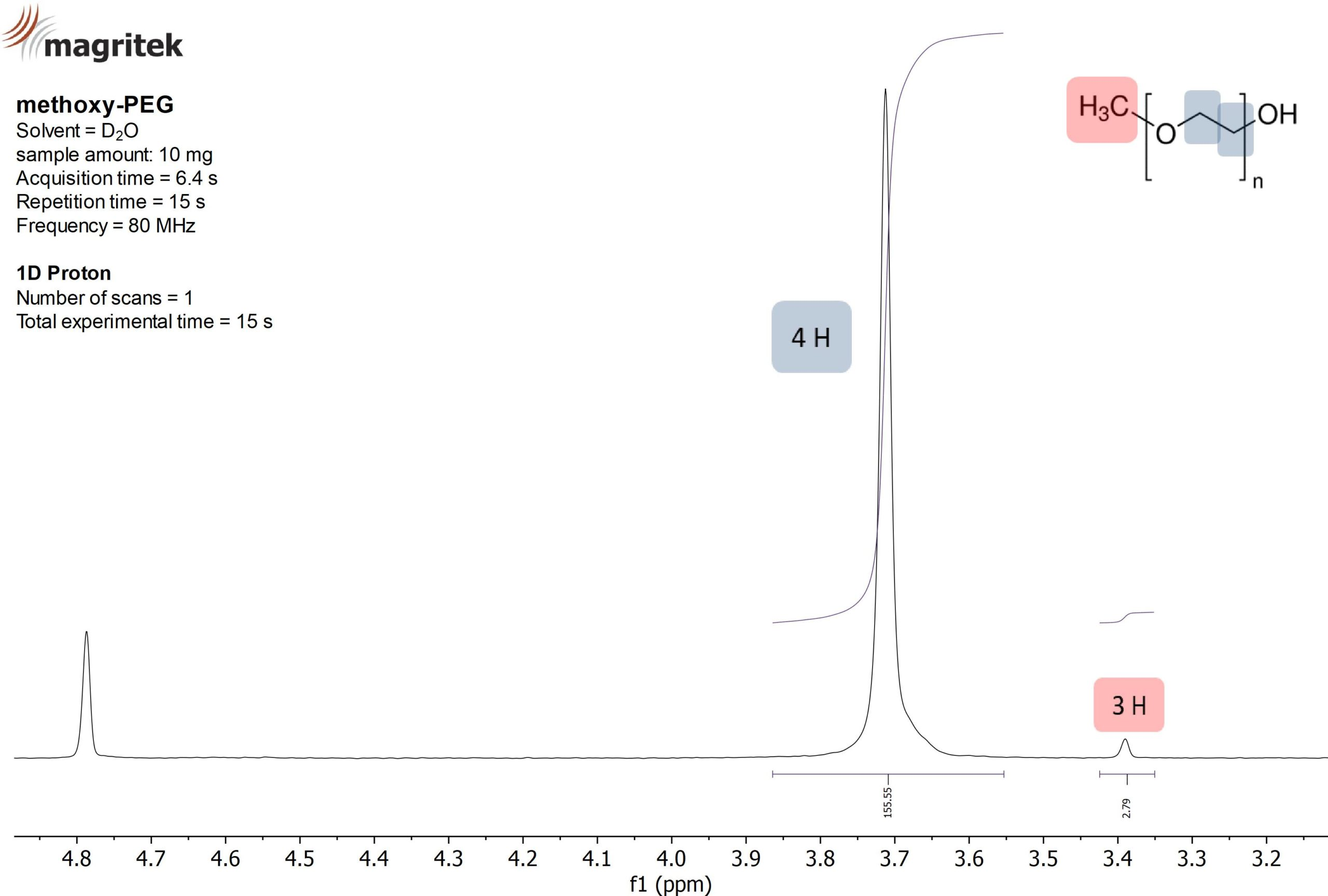Figure 1: 1D 1H spectra of polyethylene glycol (PEG)with a methoxy group acquired on a Spinsolve 80 MHz system.

The second example exhibits a  poly ε-caprolactone (Fig. 2) polymer, which is functionalized with a Tosyl end group at both ends. Functional groups are introduced specifically for the molecular weight determination or for the dedicated synthesis of copolymers and/or post functionalization. In the current case the Tosyl-function can serve all these purposes.

As the polymer is terminated at both ends with the Tosylgroup the number of protons that needs to be considered is 2×4 = 8, hence the Mn of the polymer can be calculated as follows.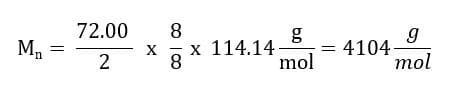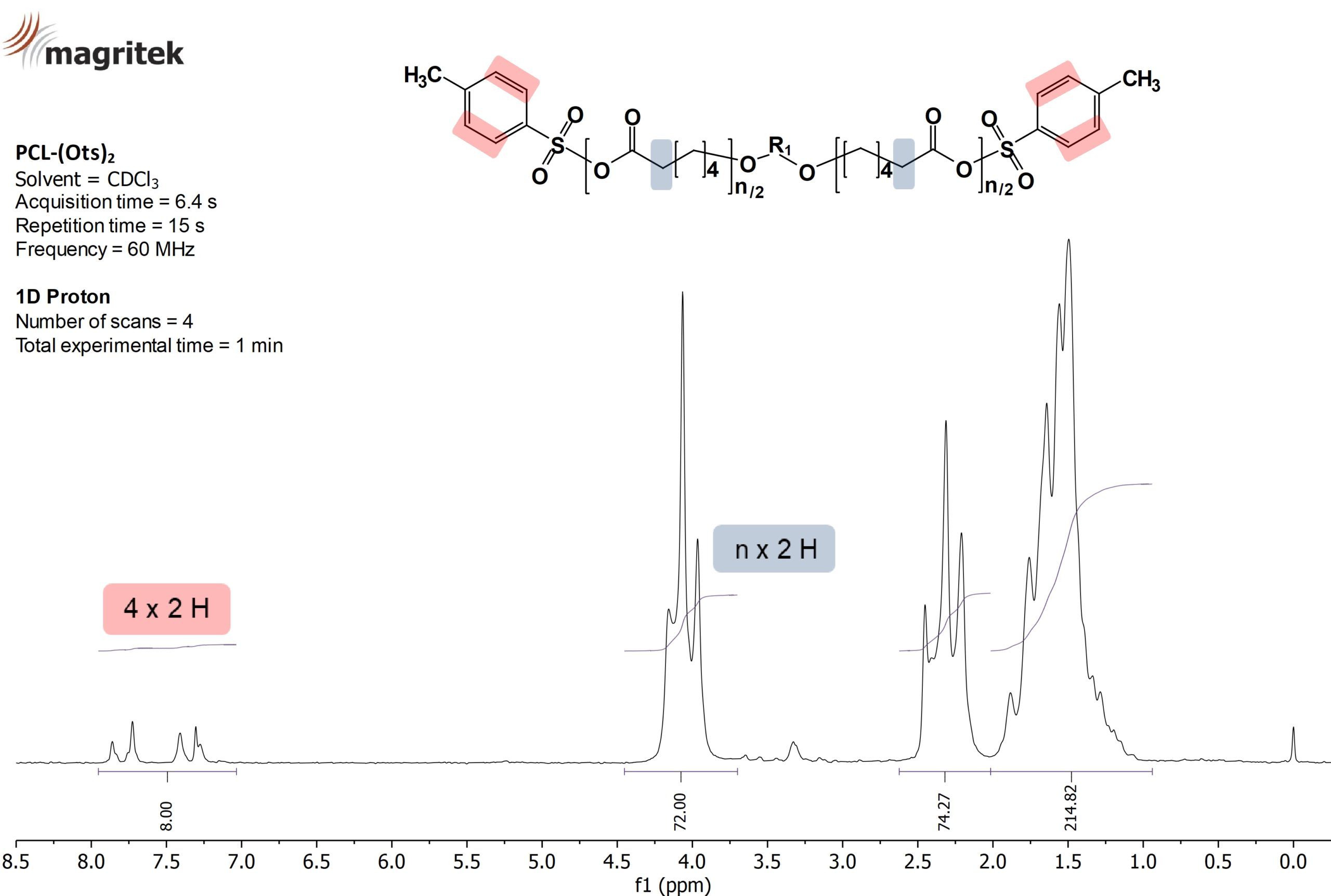Figure 2: 1D 1H spectra of poly(ε-caprolactone) end functionalized with a tosyl group on each chain end (calculated DP of 36, molecular weight of 4100 g/mol)  acquired on a Spinsolve 60 MHz. R1 is depending on the bifunctional initiator used for the polymerization.

Visit the Applications page on the www.magritek.com for more examples on how benchtop NMR can be used in different application fields.